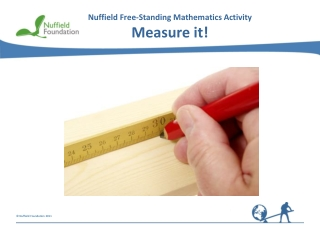DownloadDownload PresentationNuffield Free-Standing Mathematics Activity Measure it!

# Nuffield Free-Standing Mathematics Activity Measure it!

Download Presentation## Nuffield Free-Standing Mathematics Activity Measure it!

- - - - - - - - - - - - - - - - - - - - - - - - - - - E N D - - - - - - - - - - - - - - - - - - - - - - - - - - -
##### Presentation Transcript

1. Nuffield Free-Standing Mathematics ActivityMeasure it!

2. What could you use to measure these? What units would you use? This activity is about estimating and measuring lengths.

3. 0 60 30 40 50 70 10 20 80 mm 6 3 4 5 7 0 8 2 1 cm 0.4 cm 3.5 cm 6.8 cm 4 mm 68 mm 35 mm

4. 6 3 4 5 7 0 8 2 1 cm D H C E G B A F Write these measurements in centimetresandmillimetres: A = 1.5 cm B =5.1 cm = 15 mm = 51 mm C =4.4 cm D =2.3 cm = 44 mm = 23 mm E =3.6 cm F =5.9 cm = 36 mm = 59 mm G = 7.6 cm H =0.7 cm = 76 mm = 7 mm

5. How do you measure the length of a pencil? Is this correct? One end of the pencil must be at 0. Read the length on the ruler at the other end of the pencil.

6. Measure it! At the end of the activity How many centimetres are there in 1 metre? How many centimetres are there in 5 metres? How many millimetres are there in 1 metre? How many millimetres are there in 2 metres? How long is your ruler? Roughly how wide is your little finger? Roughly how long is your foot? Roughly how wide is your armspan? How many millimetres are there in 1 centimetre? How many millimetres are there in 1.5 cm? What length in centimetres is the same as 24 mm?